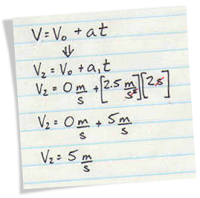1. Identify what is asked in the problem
2. Recall the concepts related to the problem and identify the formula to be used
3. List the given values in the problem
4. Make sure that only one variable is unknown
5. If more variables are unknown, try to solve them using the given values
6. Calculate the answer using the formula
7. State the answer to the problem

You cannot solve a problem if you don’t know what is the problem.

Read the physics problem carefully and slowly. Identify what the problem is all about and what it is asking. for example, consider this problem:

Michael is driving his sports car at 30 m/s when he sees a dog on the road ahead. He slams on the brakes and comes to a stop in 3.0 second. What was the acceleration of Michaels’s car?

What is asked in the problem? It is the acceleration of the car that Michael is driving.

#### Recall Concepts and Identify the Formula

Do you remember what is acceleration? It is the difference between the final and initial velocity divided by the time. Do you have the formula of acceleration in your mind?
Acceleration = Final Velocity – Initial Velocity / Time

You have to memorize these formulas or if you can’t, just be familiar with them. You will soon notice that you can remember these formulas if you keep using them.

#### List the Given Values

Things will be clear for you if you list the values given in the problem. In our sample problem above,

Initial Velocity = 30 m/s
Final Velocity = 0 m/s since the car came to a stop
Time = 3.0 s
Acceleration = unknown

Now you know that you are given with three values in the formula and the Acceleration is the only one unknown to us.

#### If More Variables are Unknown, Solve them First

This is a common problem in problem solving. For example, you might be asked to find the Torque of a rotating stick but you are only given with the mass that makes it rotate.
We know that Torque is equal to Force x Length of the lever arm. But we do not know the Force applied by the mass.
To solve this, you have to get the Force using the given mass. Force due to the weight of an object is equal to Mass x Acceleration due to gravity.

#### Solve the Problem Using the Formula

Now that you have all the variables except the one unknown, you can solve for the answer.
If you think this is the part where you have the most difficulty, I have only one tip for you:

#### State the Answer to the Problem

Although you may stop when you arrived at the answer, it is always a good practice to state the answer of the problem.
You may write:
Therefore, the acceleration of the car is -10 m/s2.